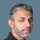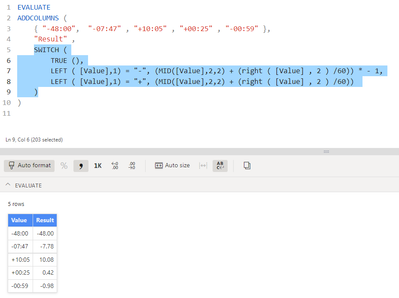cancel
Showing results for
Did you mean:

Fabric is Generally Available. Browse Fabric Presentations. Work towards your Fabric certification with the Cloud Skills Challenge.New Member

## Negative and positive time to decimal

I have a column of time duration that is formatted as text.  If I try and format the column any other way I lose my negatives.  I need to convert negative and positive time durings such as  -48:00 and -07:47 to time decimal representation such as -48.00 and -7.78.  Does anyone have any suggestions?  Thank you in advance.

2 ACCEPTED SOLUTIONSSuper User

@Daryl33609
If it works accept the asnwer as solution otherwise explain the issue/requirment

Did I answer your question? Mark my post as a solution! and hit thumbs upNew Member

Ignore my prior post.  I made a few more tweaks to your code and got it to work.  Thank you so much for getting me past this issue.  Here is my code:

Column 2 = SWITCH (

TRUE (),

LEFT ( [Actual Total Hours (Include Corrections)],1) = "-", (MID([Actual Total Hours (Include Corrections)],2,2) + (right ( [Actual Total Hours (Include Corrections)] , 2 ) /60)) * - 1,

LEFT ( [Actual Total Hours (Include Corrections)],1) <> "-", (MID([Actual Total Hours (Include Corrections)],1,2) + (right ( [Actual Total Hours (Include Corrections)] , 2 ) /60)))

5 REPLIES 5Super User

@Daryl33609

You can add a column as follows. I assume you have + and - for each value

``````Col =
SWITCH (
TRUE (),
LEFT ( [Value],1) = "-", (MID([Value],2,2) + (right ( [Value] , 2 ) /60)) * - 1,
LEFT ( [Value],1) = "+", (MID([Value],2,2) + (right ( [Value] , 2 ) /60))
)``````Did I answer your question? Mark my post as a solution! and hit thumbs upNew Member

I am very close, thanks to your script.  The negative transformation is working, but not the positive.  Here is my code:

Column 2 = SWITCH (
TRUE (),
LEFT ( [Actual Total Hours (Include Corrections)],1) = "-", (MID([Actual Total Hours (Include Corrections)],2,2) + (right ( [Actual Total Hours (Include Corrections)] , 2 ) /60)) * - 1,
LEFT ( [Actual Total Hours (Include Corrections)],1) = "+", (MID([Actual Total Hours (Include Corrections)],2,2) + (right ( [Actual Total Hours (Include Corrections)] , 2 ) /60)))Any idea why the positive time is not converting?New Member

Thank you so much.  I will try this!Super User

@Daryl33609
If it works accept the asnwer as solution otherwise explain the issue/requirment

Did I answer your question? Mark my post as a solution! and hit thumbs upNew Member

Ignore my prior post.  I made a few more tweaks to your code and got it to work.  Thank you so much for getting me past this issue.  Here is my code:

Column 2 = SWITCH (

TRUE (),

LEFT ( [Actual Total Hours (Include Corrections)],1) = "-", (MID([Actual Total Hours (Include Corrections)],2,2) + (right ( [Actual Total Hours (Include Corrections)] , 2 ) /60)) * - 1,

LEFT ( [Actual Total Hours (Include Corrections)],1) <> "-", (MID([Actual Total Hours (Include Corrections)],1,2) + (right ( [Actual Total Hours (Include Corrections)] , 2 ) /60)))Announcements#### Power BI Monthly Update - November 2023

Check out the November 2023 Power BI update to learn about new features.#### Fabric Community News unified experience

Read the latest Fabric Community announcements, including updates on Power BI, Synapse, Data Factory and Data Activator.#### The largest Power BI and Fabric virtual conference

130+ sessions, 130+ speakers, Product managers, MVPs, and experts. All about Power BI and Fabric. Attend online or watch the recordings.Top Solution Authors
Top Kudoed Authors
Users online (2,210)